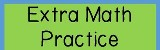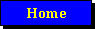NallPro's Math Help Point of View Extra     WorksheetsOnly 4 NallPro => Ask Math Question MATH for Kids Multiplication-Table Memorize Multiplication Metric Measurement Geometry Help - I Geometry Help - II Algebra Formulas Shapes Formula - I Shapes Formula - II Arithmetic Formula Geometric Formula Trigonometric Identities Tri. Exact Angle Trigonometric Identities Derivative Formulas Higher-Derivative Hyperbolic Functions Integral Formulas Complex Numbers 3D-Lines Formulas Sample Worksheets! Mathematicians MATH Just for Fun FAQs  [1stClick then call] Register Online -> Contact Us